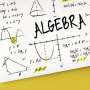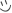# How good are you at algebra?Ah...algebra. Some love it, some loathe it. You've most likely interacted with this subject quite a bit in your education. I personally have been out of the algebraic universe for over three years, so I made this little quiz to sharpen my skills in this rich subject.

This quiz will cover some of the basic concepts of algebra. In case you don't already know (and if you're actually reading this!), a "^" symbol indicates an exponent, and a "/" indicates division. Good luck! Make sure to grab a pencil and paper, because you may need them for some of the problems.

Created by: Hiccstrid of tumblr

1. Factor 7x - 56.
2. Factor 2x + 3y.
3. Factor (x^2) - 5x.
4. Factor (x^2) - 2x + 1.
5. Factor (x^2) + 11x + 28.
6. Factor 5(x^2) + 7x - 24.
7. Simplify 4x + 7y - 5x + 2y.
8. Simplify x + 4x - 2.
9. Simplify 3x - 2(x^2) + 4x - 7.
10. Simplify 3(x^3) - 5(y^2) - 2x + (x^2) - 3(y^2) + 4y - 2(x^2) + (y^2) + 2(x^3) - y.
11. x - 4 = 9. Solve for x.
12. 7 - x = -2. Solve for x.
13. 5 = x + 3. Solve for x.
14. 14 + x = 8
15. 3x - 7 = 29. Solve for x.
16. 1 + 2x = 7. Solve for x.
17. 5y - 7x = 10. Solve for x.
18. x + y = 11; 4x - y = 4. Solve for x and y
19. y - x = -2; x + y = 12. Solve for x and y.
20. 5xy = -10; (y/x) = -2. Solve for x and y.

You're about to get your result. Then try our new sharing options.